# Orchestrate Jobs to Train and Evaluate Models with Amazon SageMaker Pipelines

This notebook’s CI test result for us-west-2 is as follows. CI test results in other regions can be found at the end of the notebook.Amazon SageMaker Pipelines offers machine learning (ML) application developers and operations engineers the ability to orchestrate SageMaker jobs and author reproducible ML pipelines. It also enables them to deploy custom-built models for inference in real-time with low latency, run offline inferences with Batch Transform, and track lineage of artifacts. They can institute sound operational practices in deploying and monitoring production workflows, deploying model artifacts, and tracking artifact lineage through a simple interface, adhering to safety and best practice paradigms for ML application development.

The SageMaker Pipelines service supports a SageMaker Pipeline domain specific language (DSL), which is a declarative JSON specification. This DSL defines a directed acyclic graph (DAG) of pipeline parameters and SageMaker job steps. The SageMaker Python Software Developer Kit (SDK) streamlines the generation of the pipeline DSL using constructs that engineers and scientists are already familiar with.

## Runtime

This notebook takes approximately an hour to run.

## SageMaker Pipelines

SageMaker Pipelines supports the following activities, which are demonstrated in this notebook:

• Pipelines - A DAG of steps and conditions to orchestrate SageMaker jobs and resource creation.

• Processing job steps - A simplified, managed experience on SageMaker to run data processing workloads, such as feature engineering, data validation, model evaluation, and model interpretation.

• Training job steps - An iterative process that teaches a model to make predictions by presenting examples from a training dataset.

• Conditional execution steps - A step that provides conditional execution of branches in a pipeline.

• Register model steps - A step that creates a model package resource in the Model Registry that can be used to create deployable models in Amazon SageMaker.

• Create model steps - A step that creates a model for use in transform steps or later publication as an endpoint.

• Transform job steps - A batch transform to preprocess datasets to remove noise or bias that interferes with training or inference from a dataset, get inferences from large datasets, and run inference when a persistent endpoint is not needed.

• Fail steps - A step that stops a pipeline execution and marks the pipeline execution as failed.

• Parametrized Pipeline executions - Enables variation in pipeline executions according to specified parameters.

## Notebook Overview

This notebook shows how to:

• Define a set of Pipeline parameters that can be used to parametrize a SageMaker Pipeline.

• Define a Processing step that performs cleaning, feature engineering, and splitting the input data into train and test data sets.

• Define a Training step that trains a model on the preprocessed train data set.

• Define a Processing step that evaluates the trained model’s performance on the test dataset.

• Define a Create Model step that creates a model from the model artifacts used in training.

• Define a Transform step that performs batch transformation based on the model that was created.

• Define a Register Model step that creates a model package from the estimator and model artifacts used to train the model.

• Define a Conditional step that measures a condition based on output from prior steps and conditionally executes other steps.

• Define a Fail step with a customized error message indicating the cause of the execution failure.

• Define and create a Pipeline definition in a DAG, with the defined parameters and steps.

• Start a Pipeline execution and wait for execution to complete.

• Download the model evaluation report from the S3 bucket for examination.

• Start a second Pipeline execution.

## A SageMaker Pipeline

The pipeline that you create follows a typical machine learning (ML) application pattern of preprocessing, training, evaluation, model creation, batch transformation, and model registration:## Dataset

The dataset you use is the UCI Machine Learning Abalone Dataset . The aim for this task is to determine the age of an abalone snail from its physical measurements. At the core, this is a regression problem.

The dataset contains several features: length (the longest shell measurement), diameter (the diameter perpendicular to length), height (the height with meat in the shell), whole_weight (the weight of whole abalone), shucked_weight (the weight of meat), viscera_weight (the gut weight after bleeding), shell_weight (the weight after being dried), sex (‘M’, ‘F’, ‘I’ where ‘I’ is Infant), and rings (integer).

The number of rings turns out to be a good approximation for age (age is rings + 1.5). However, to obtain this number requires cutting the shell through the cone, staining the section, and counting the number of rings through a microscope, which is a time-consuming task. However, the other physical measurements are easier to determine. You use the dataset to build a predictive model of the variable rings through these other physical measurements.

Before you upload the data to an S3 bucket, install the SageMaker Python SDK and gather some constants you can use later in this notebook.

 Dua, D. and Graff, C. (2019). UCI Machine Learning Repository. Irvine, CA: University of California, School of Information and Computer Science.

[ ]:

!pip install -U sagemaker

[ ]:

import sys

import boto3
import sagemaker
from sagemaker.workflow.pipeline_context import PipelineSession

sagemaker_session = sagemaker.session.Session()
region = sagemaker_session.boto_region_name
role = sagemaker.get_execution_role()
pipeline_session = PipelineSession()
default_bucket = sagemaker_session.default_bucket()
model_package_group_name = f"AbaloneModelPackageGroupName"


Now, upload the data into the default bucket. You can select our own data set for the input_data_uri as is appropriate.

[ ]:

!mkdir -p data

[ ]:

local_path = "data/abalone-dataset.csv"

s3 = boto3.resource("s3")
"datasets/tabular/uci_abalone/abalone.csv", local_path
)

base_uri = f"s3://{default_bucket}/abalone"
local_path=local_path,
desired_s3_uri=base_uri,
)
print(input_data_uri)


Download a second dataset for batch transformation after model creation. You can select our own dataset for the batch_data_uri as is appropriate.

[ ]:

local_path = "data/abalone-dataset-batch"

s3 = boto3.resource("s3")
"dataset/abalone-dataset-batch", local_path
)

base_uri = f"s3://{default_bucket}/abalone"
local_path=local_path,
desired_s3_uri=base_uri,
)
print(batch_data_uri)


## Define Parameters to Parametrize Pipeline Execution

Define Pipeline parameters that you can use to parametrize the pipeline. Parameters enable custom pipeline executions and schedules without having to modify the Pipeline definition.

The supported parameter types include:

• ParameterString - represents a str Python type

• ParameterInteger - represents an int Python type

• ParameterFloat - represents a float Python type

These parameters support providing a default value, which can be overridden on pipeline execution. The default value specified should be an instance of the type of the parameter.

The parameters defined in this workflow include:

• processing_instance_count - The instance count of the processing job.

• instance_type - The ml.* instance type of the training job.

• model_approval_status - The approval status to register with the trained model for CI/CD purposes (“PendingManualApproval” is the default).

• input_data - The S3 bucket URI location of the input data.

• batch_data - The S3 bucket URI location of the batch data.

• mse_threshold - The Mean Squared Error (MSE) threshold used to verify the accuracy of a model.

[ ]:

from sagemaker.workflow.parameters import (
ParameterInteger,
ParameterString,
ParameterFloat,
)

processing_instance_count = ParameterInteger(name="ProcessingInstanceCount", default_value=1)
instance_type = ParameterString(name="TrainingInstanceType", default_value="ml.m5.xlarge")
model_approval_status = ParameterString(
name="ModelApprovalStatus", default_value="PendingManualApproval"
)
input_data = ParameterString(
name="InputData",
default_value=input_data_uri,
)
batch_data = ParameterString(
name="BatchData",
default_value=batch_data_uri,
)
mse_threshold = ParameterFloat(name="MseThreshold", default_value=6.0)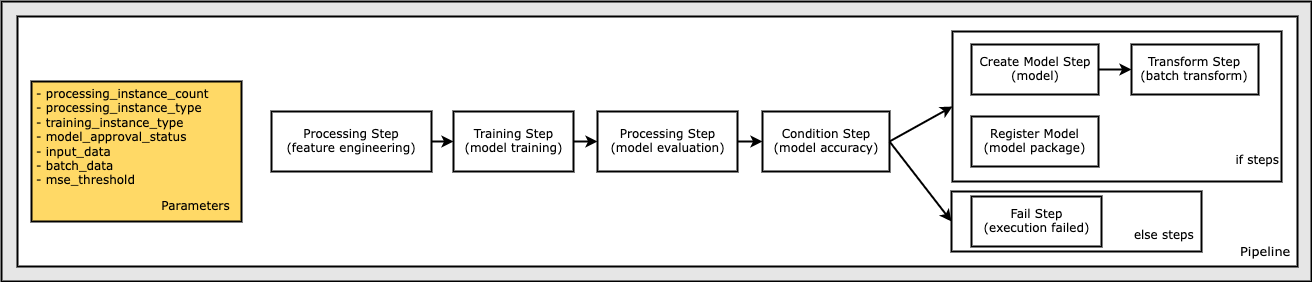## Define a Processing Step for Feature Engineering

First, develop a preprocessing script that is specified in the Processing step.

This notebook cell writes a file preprocessing_abalone.py, which contains the preprocessing script. You can update the script, and rerun this cell to overwrite. The preprocessing script uses scikit-learn to do the following:

• Fill in missing sex category data and encode it so that it is suitable for training.

• Scale and normalize all numerical fields, aside from sex and rings numerical data.

• Split the data into training, validation, and test datasets.

The Processing step executes the script on the input data. The Training step uses the preprocessed training features and labels to train a model. The Evaluation step uses the trained model and preprocessed test features and labels to evaluate the model.

[ ]:

!mkdir -p code

[ ]:

%%writefile code/preprocessing.py
import argparse
import os
import requests
import tempfile

import numpy as np
import pandas as pd

from sklearn.compose import ColumnTransformer
from sklearn.impute import SimpleImputer
from sklearn.pipeline import Pipeline
from sklearn.preprocessing import StandardScaler, OneHotEncoder

# Since we get a headerless CSV file, we specify the column names here.
feature_columns_names = [
"sex",
"length",
"diameter",
"height",
"whole_weight",
"shucked_weight",
"viscera_weight",
"shell_weight",
]
label_column = "rings"

feature_columns_dtype = {
"sex": str,
"length": np.float64,
"diameter": np.float64,
"height": np.float64,
"whole_weight": np.float64,
"shucked_weight": np.float64,
"viscera_weight": np.float64,
"shell_weight": np.float64,
}
label_column_dtype = {"rings": np.float64}

def merge_two_dicts(x, y):
z = x.copy()
z.update(y)
return z

if __name__ == "__main__":
base_dir = "/opt/ml/processing"

f"{base_dir}/input/abalone-dataset.csv",
names=feature_columns_names + [label_column],
dtype=merge_two_dicts(feature_columns_dtype, label_column_dtype),
)
numeric_features = list(feature_columns_names)
numeric_features.remove("sex")
numeric_transformer = Pipeline(
steps=[("imputer", SimpleImputer(strategy="median")), ("scaler", StandardScaler())]
)

categorical_features = ["sex"]
categorical_transformer = Pipeline(
steps=[
("imputer", SimpleImputer(strategy="constant", fill_value="missing")),
("onehot", OneHotEncoder(handle_unknown="ignore")),
]
)

preprocess = ColumnTransformer(
transformers=[
("num", numeric_transformer, numeric_features),
("cat", categorical_transformer, categorical_features),
]
)

y = df.pop("rings")
X_pre = preprocess.fit_transform(df)
y_pre = y.to_numpy().reshape(len(y), 1)

X = np.concatenate((y_pre, X_pre), axis=1)

np.random.shuffle(X)
train, validation, test = np.split(X, [int(0.7 * len(X)), int(0.85 * len(X))])

pd.DataFrame(validation).to_csv(
)


Next, create an instance of a SKLearnProcessor processor and use that in our ProcessingStep.

You also specify the framework_version to use throughout this notebook.

Note the processing_instance_count parameter used by the processor instance.

[ ]:

from sagemaker.sklearn.processing import SKLearnProcessor

framework_version = "1.2-1"

sklearn_processor = SKLearnProcessor(
framework_version=framework_version,
instance_type="ml.m5.xlarge",
instance_count=processing_instance_count,
base_job_name="sklearn-abalone-process",
role=role,
sagemaker_session=pipeline_session,
)


Finally, we take the output of the processor’s run method and pass that as arguments to the ProcessingStep. By passing the pipeline_session to the sagemaker_session, calling .run() does not launch the processing job, it returns the arguments needed to run the job as a step in the pipeline.

Note the "train_data" and "test_data" named channels specified in the output configuration for the processing job. Step Properties can be used in subsequent steps and resolve to their runtime values at execution. Specifically, this usage is called out when you define the training step.

[ ]:

from sagemaker.processing import ProcessingInput, ProcessingOutput
from sagemaker.workflow.steps import ProcessingStep

processor_args = sklearn_processor.run(
inputs=[
ProcessingInput(source=input_data, destination="/opt/ml/processing/input"),
],
outputs=[
ProcessingOutput(output_name="train", source="/opt/ml/processing/train"),
ProcessingOutput(output_name="validation", source="/opt/ml/processing/validation"),
ProcessingOutput(output_name="test", source="/opt/ml/processing/test"),
],
code="code/preprocessing.py",
)

step_process = ProcessingStep(name="AbaloneProcess", step_args=processor_args)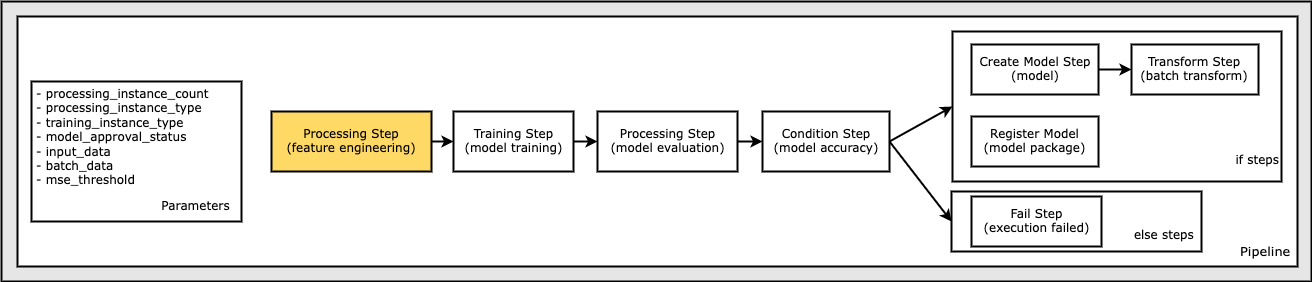## Define a Training Step to Train a Model

In this section, use Amazon SageMaker’s XGBoost Algorithm to train on this dataset. Configure an Estimator for the XGBoost algorithm and the input dataset. A typical training script loads data from the input channels, configures training with hyperparameters, trains a model, and saves a model to model_dir so that it can be hosted later.

The model path where the models from training are saved is also specified.

Note the instance_type parameter may be used in multiple places in the pipeline. In this case, the instance_type is passed into the estimator.

[ ]:

from sagemaker.estimator import Estimator
from sagemaker.inputs import TrainingInput

model_path = f"s3://{default_bucket}/AbaloneTrain"
image_uri = sagemaker.image_uris.retrieve(
framework="xgboost",
region=region,
version="1.0-1",
py_version="py3",
instance_type="ml.m5.xlarge",
)
xgb_train = Estimator(
image_uri=image_uri,
instance_type=instance_type,
instance_count=1,
output_path=model_path,
role=role,
sagemaker_session=pipeline_session,
)
xgb_train.set_hyperparameters(
objective="reg:linear",
num_round=50,
max_depth=5,
eta=0.2,
gamma=4,
min_child_weight=6,
subsample=0.7,
)

train_args = xgb_train.fit(
inputs={
"train": TrainingInput(
s3_data=step_process.properties.ProcessingOutputConfig.Outputs["train"].S3Output.S3Uri,
content_type="text/csv",
),
"validation": TrainingInput(
s3_data=step_process.properties.ProcessingOutputConfig.Outputs[
"validation"
].S3Output.S3Uri,
content_type="text/csv",
),
}
)


Finally, we use the output of the estimator’s .fit() method as arguments to the TrainingStep. By passing the pipeline_session to the sagemaker_session, calling .fit() does not launch the training job, it returns the arguments needed to run the job as a step in the pipeline.

Pass in the S3Uri of the "train_data" output channel to the .fit() method. Also, use the other "test_data" output channel for model evaluation in the pipeline. The properties attribute of a Pipeline step matches the object model of the corresponding response of a describe call. These properties can be referenced as placeholder values and are resolved at runtime. For example, the ProcessingStep properties attribute matches the object model of the DescribeProcessingJob response object.

[ ]:

from sagemaker.inputs import TrainingInput
from sagemaker.workflow.steps import TrainingStep

step_train = TrainingStep(
name="AbaloneTrain",
step_args=train_args,
)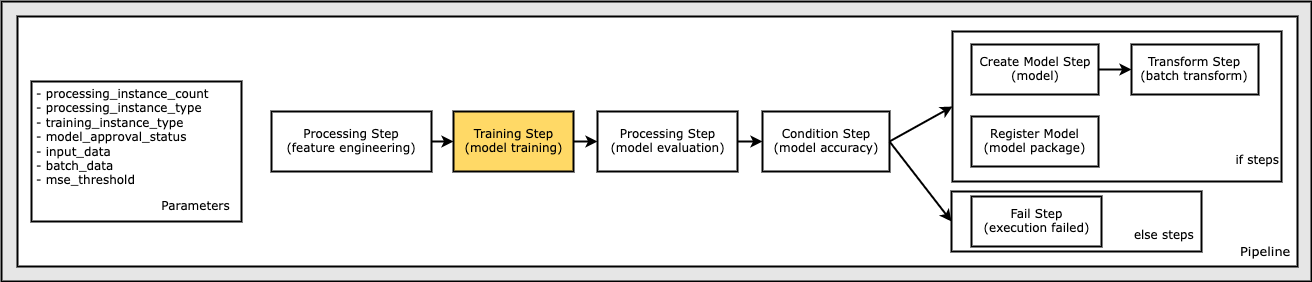## Define a Model Evaluation Step to Evaluate the Trained Model

First, develop an evaluation script that is specified in a Processing step that performs the model evaluation.

After pipeline execution, you can examine the resulting evaluation.json for analysis.

The evaluation script uses xgboost to do the following:

• Issue predictions against the test data.

• Build a classification report, including accuracy and ROC curve.

• Save the evaluation report to the evaluation directory.

[ ]:

%%writefile code/evaluation.py
import json
import pathlib
import pickle
import tarfile

import joblib
import numpy as np
import pandas as pd
import xgboost

from sklearn.metrics import mean_squared_error

if __name__ == "__main__":
model_path = f"/opt/ml/processing/model/model.tar.gz"
with tarfile.open(model_path) as tar:
tar.extractall(path=".")

test_path = "/opt/ml/processing/test/test.csv"

y_test = df.iloc[:, 0].to_numpy()
df.drop(df.columns, axis=1, inplace=True)

X_test = xgboost.DMatrix(df.values)

predictions = model.predict(X_test)

mse = mean_squared_error(y_test, predictions)
std = np.std(y_test - predictions)
report_dict = {
"regression_metrics": {
"mse": {"value": mse, "standard_deviation": std},
},
}

output_dir = "/opt/ml/processing/evaluation"
pathlib.Path(output_dir).mkdir(parents=True, exist_ok=True)

evaluation_path = f"{output_dir}/evaluation.json"
with open(evaluation_path, "w") as f:
f.write(json.dumps(report_dict))


Next, create an instance of a ScriptProcessor processor and use it in the ProcessingStep.

[ ]:

from sagemaker.processing import ScriptProcessor

script_eval = ScriptProcessor(
image_uri=image_uri,
command=["python3"],
instance_type="ml.m5.xlarge",
instance_count=1,
base_job_name="script-abalone-eval",
role=role,
sagemaker_session=pipeline_session,
)

eval_args = script_eval.run(
inputs=[
ProcessingInput(
source=step_train.properties.ModelArtifacts.S3ModelArtifacts,
destination="/opt/ml/processing/model",
),
ProcessingInput(
source=step_process.properties.ProcessingOutputConfig.Outputs["test"].S3Output.S3Uri,
destination="/opt/ml/processing/test",
),
],
outputs=[
ProcessingOutput(output_name="evaluation", source="/opt/ml/processing/evaluation"),
],
code="code/evaluation.py",
)


Use the processor’s arguments returned by .run() to construct a ProcessingStep, along with the input and output channels and the code that will be executed when the pipeline invokes pipeline execution.

Specifically, the S3ModelArtifacts from the step_train properties and the S3Uri of the "test_data" output channel of the step_process properties are passed as inputs. The TrainingStep and ProcessingStep properties attribute matches the object model of the DescribeTrainingJob and DescribeProcessingJob response objects, respectively.

[ ]:

from sagemaker.workflow.properties import PropertyFile

evaluation_report = PropertyFile(
name="EvaluationReport", output_name="evaluation", path="evaluation.json"
)
step_eval = ProcessingStep(
name="AbaloneEval",
step_args=eval_args,
property_files=[evaluation_report],
)## Define a Create Model Step to Create a Model

In order to perform batch transformation using the example model, create a SageMaker model.

Specifically, pass in the S3ModelArtifacts from the TrainingStep, step_train properties. The TrainingStep properties attribute matches the object model of the DescribeTrainingJob response object.

[ ]:

from sagemaker.model import Model

model = Model(
image_uri=image_uri,
model_data=step_train.properties.ModelArtifacts.S3ModelArtifacts,
sagemaker_session=pipeline_session,
role=role,
)


Define the ModelStep by providing the return values from model.create() as the step arguments.

[ ]:

from sagemaker.inputs import CreateModelInput
from sagemaker.workflow.model_step import ModelStep

step_create_model = ModelStep(
name="AbaloneCreateModel",
step_args=model.create(instance_type="ml.m5.large", accelerator_type="ml.eia1.medium"),
)


## Define a Transform Step to Perform Batch Transformation

Now that a model instance is defined, create a Transformer instance with the appropriate model type, compute instance type, and desired output S3 URI.

Specifically, pass in the ModelName from the CreateModelStep, step_create_model properties. The CreateModelStep properties attribute matches the object model of the DescribeModel response object.

[ ]:

from sagemaker.transformer import Transformer

transformer = Transformer(
model_name=step_create_model.properties.ModelName,
instance_type="ml.m5.xlarge",
instance_count=1,
output_path=f"s3://{default_bucket}/AbaloneTransform",
)


Pass in the transformer instance and the TransformInput with the batch_data pipeline parameter defined earlier.

[ ]:

from sagemaker.inputs import TransformInput
from sagemaker.workflow.steps import TransformStep

step_transform = TransformStep(
name="AbaloneTransform", transformer=transformer, inputs=TransformInput(data=batch_data)
)


## Define a Register Model Step to Create a Model Package

A model package is an abstraction of reusable model artifacts that packages all ingredients required for inference. Primarily, it consists of an inference specification that defines the inference image to use along with an optional model weights location.

A model package group is a collection of model packages. A model package group can be created for a specific ML business problem, and new versions of the model packages can be added to it. Typically, customers are expected to create a ModelPackageGroup for a SageMaker pipeline so that model package versions can be added to the group for every SageMaker Pipeline run.

To register a model in the Model Registry, we take the model created in the previous steps

model = Model(
image_uri=image_uri,
model_data=step_train.properties.ModelArtifacts.S3ModelArtifacts,
sagemaker_session=pipeline_session,
role=role,
)


and call the .register() function on it while passing all the parameters needed for registering the model.

We take the outputs of the .register() call and pass that to the ModelStep as step arguments.

[ ]:

from sagemaker.model_metrics import MetricsSource, ModelMetrics

model_metrics = ModelMetrics(
model_statistics=MetricsSource(
s3_uri="{}/evaluation.json".format(
step_eval.arguments["ProcessingOutputConfig"]["Outputs"]["S3Output"]["S3Uri"]
),
content_type="application/json",
)
)

register_args = model.register(
content_types=["text/csv"],
response_types=["text/csv"],
inference_instances=["ml.t2.medium", "ml.m5.xlarge"],
transform_instances=["ml.m5.xlarge"],
model_package_group_name=model_package_group_name,
approval_status=model_approval_status,
model_metrics=model_metrics,
)
step_register = ModelStep(name="AbaloneRegisterModel", step_args=register_args)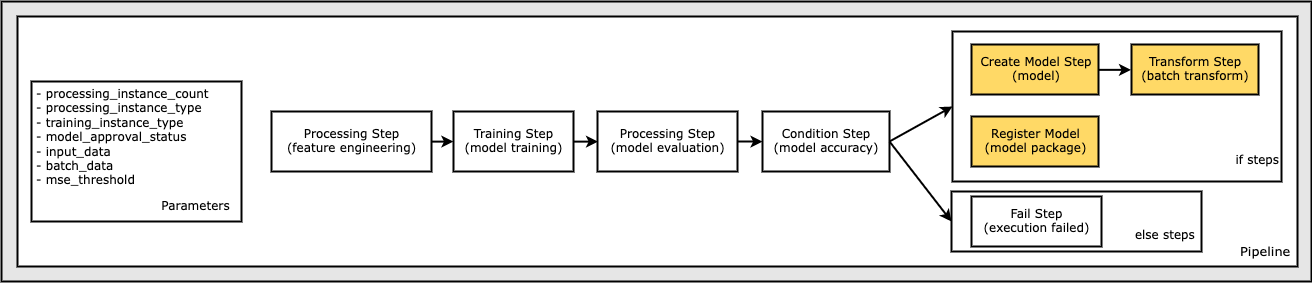## Define a Fail Step to Terminate the Pipeline Execution and Mark it as Failed

This section walks you through the following steps:

• Define a FailStep with customized error message, which indicates the cause of the execution failure.

• Enter the FailStep error message with a Join function, which appends a static text string with the dynamic mse_threshold parameter to build a more informative error message.

[ ]:

from sagemaker.workflow.fail_step import FailStep
from sagemaker.workflow.functions import Join

step_fail = FailStep(
name="AbaloneMSEFail",
error_message=Join(on=" ", values=["Execution failed due to MSE >", mse_threshold]),
)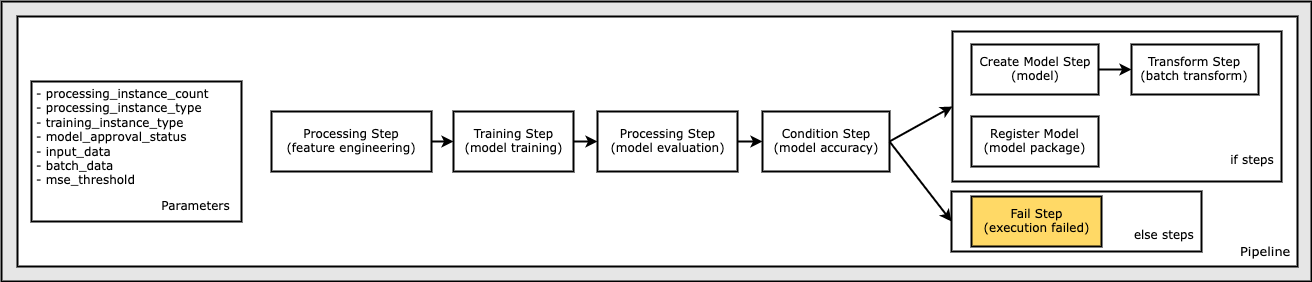## Define a Condition Step to Check Accuracy and Conditionally Create a Model and Run a Batch Transformation and Register a Model in the Model Registry, Or Terminate the Execution in Failed State

In this step, the model is registered only if the accuracy of the model, as determined by the evaluation step step_eval, exceeded a specified value. Otherwise, the pipeline execution fails and terminates. A ConditionStep enables pipelines to support conditional execution in the pipeline DAG based on the conditions of the step properties.

In the following section, you:

• Define a ConditionLessThanOrEqualTo on the accuracy value found in the output of the evaluation step, step_eval.

• Use the condition in the list of conditions in a ConditionStep.

• Pass the CreateModelStep and TransformStep steps, and the RegisterModel step collection into the if_steps of the ConditionStep, which are only executed if the condition evaluates to True.

• Pass the FailStep step into the else_stepsof the ConditionStep, which is only executed if the condition evaluates to False.

[ ]:

from sagemaker.workflow.conditions import ConditionLessThanOrEqualTo
from sagemaker.workflow.condition_step import ConditionStep
from sagemaker.workflow.functions import JsonGet

cond_lte = ConditionLessThanOrEqualTo(
left=JsonGet(
step_name=step_eval.name,
property_file=evaluation_report,
json_path="regression_metrics.mse.value",
),
right=mse_threshold,
)

step_cond = ConditionStep(
name="AbaloneMSECond",
conditions=[cond_lte],
if_steps=[step_register, step_create_model, step_transform],
else_steps=[step_fail],
)## Define a Pipeline of Parameters, Steps, and Conditions

In this section, combine the steps into a Pipeline so it can be executed.

A pipeline requires a name, parameters, and steps. Names must be unique within an (account, region) pair.

Note:

• All the parameters used in the definitions must be present.

• Steps passed into the pipeline do not have to be listed in the order of execution. The SageMaker Pipeline service resolves the data dependency DAG as steps for the execution to complete.

• Steps must be unique to across the pipeline step list and all condition step if/else lists.

[ ]:

from sagemaker.workflow.pipeline import Pipeline

pipeline_name = f"AbalonePipeline"
pipeline = Pipeline(
name=pipeline_name,
parameters=[
processing_instance_count,
instance_type,
model_approval_status,
input_data,
batch_data,
mse_threshold,
],
steps=[step_process, step_train, step_eval, step_cond],
)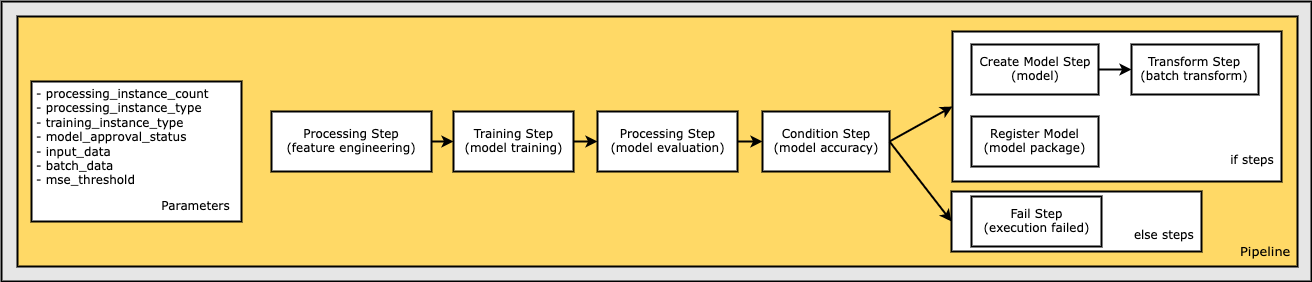### (Optional) Examining the pipeline definition

The JSON of the pipeline definition can be examined to confirm the pipeline is well-defined and the parameters and step properties resolve correctly.

[ ]:

import json

definition


## Submit the pipeline to SageMaker and start execution

Submit the pipeline definition to the Pipeline service. The Pipeline service uses the role that is passed in to create all the jobs defined in the steps.

[ ]:

pipeline.upsert(role_arn=role)


Start the pipeline and accept all the default parameters.

[ ]:

execution = pipeline.start()


## Pipeline Operations: Examining and Waiting for Pipeline Execution

Describe the pipeline execution.

[ ]:

execution.describe()


Wait for the execution to complete.

[ ]:

execution.wait()


List the steps in the execution. These are the steps in the pipeline that have been resolved by the step executor service.

[ ]:

execution.list_steps()


### Examining the Evaluation

Examine the resulting model evaluation after the pipeline completes. Download the resulting evaluation.json file from S3 and print the report.

[ ]:

from pprint import pprint

"{}/evaluation.json".format(
step_eval.arguments["ProcessingOutputConfig"]["Outputs"]["S3Output"]["S3Uri"]
)
)


### Lineage

Review the lineage of the artifacts generated by the pipeline.

[ ]:

import time
from sagemaker.lineage.visualizer import LineageTableVisualizer

viz = LineageTableVisualizer(sagemaker.session.Session())
for execution_step in reversed(execution.list_steps()):
print(execution_step)
display(viz.show(pipeline_execution_step=execution_step))
time.sleep(5)


### Parametrized Executions

You can run additional executions of the pipeline and specify different pipeline parameters. The parameters argument is a dictionary containing parameter names, and where the values are used to override the defaults values.

Based on the performance of the model, you might want to kick off another pipeline execution on a compute-optimized instance type and set the model approval status to “Approved” automatically. This means that the model package version generated by the RegisterModel step is automatically ready for deployment through CI/CD pipelines, such as with SageMaker Projects.

[ ]:

execution = pipeline.start(
parameters=dict(
ModelApprovalStatus="Approved",
)
)

[ ]:

execution.wait()

[ ]:

execution.list_steps()


Apart from that, you might also want to adjust the MSE threshold to a smaller value and raise the bar for the accuracy of the registered model. In this case you can override the MSE threshold like the following:

[ ]:

execution = pipeline.start(parameters=dict(MseThreshold=3.0))


If the MSE threshold is not satisfied, the pipeline execution enters the FailStep and is marked as failed.

[ ]:

try:
execution.wait()
except Exception as error:
print(error)

[ ]:

execution.list_steps()


## Notebook CI Test Results

This notebook was tested in multiple regions. The test results are as follows, except for us-west-2 which is shown at the top of the notebook.